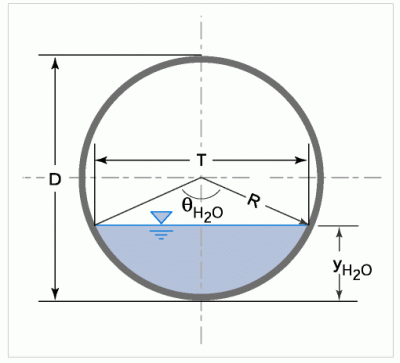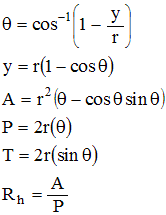The following equations are used in FishXing to calculate the wetted perimeter, top width, and cross-sectional area of the flow through a circular culvert that is not-embedded.

Definition sketch for Geometry of a circular culvert:Equations Used in the Calculation of Circular Culvert Geometry:Where:

d = Diameter of culvert

y = Depth of water in culvert

P = Wetted Perimeter of water on the bottom

T = Top width of water surface

A = Cross sectional area of flow